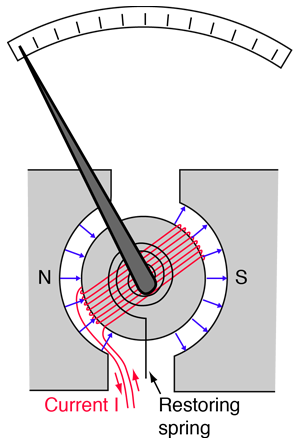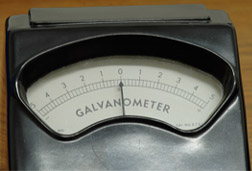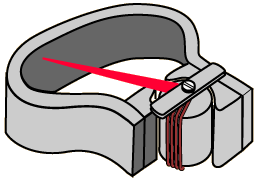# GalvanometerGalvanometer is the historical name given to a moving coil electric current detector. When a current is passed through a coil in a magnetic field, the coil experiences a torque proportional to the current. If the coil's movement is opposed by a coil spring, then the amount of deflection of a needle attached to the coil may be proportional to the current passing through the coil. Such "meter movements" were at the heart of the moving coil meters such as voltmeters and ammeters until they were largely replaced with solid state meters.The accuracy of moving coil meters is dependent upon having a uniform and constant magnetic field. The illustration shows one configuration of permanent magnet which was widely used in such meters.
 Moving coil meters
Index

DC Circuits

Magnetic force concepts

 HyperPhysics***** Electricity and Magnetism R Nave
Go Back